# 7.6: Polygons

•• Amy Lagusker
• College of the Canyons
$$\newcommand{\vecs}{\overset { \rightharpoonup} {\mathbf{#1}} }$$ $$\newcommand{\vecd}{\overset{-\!-\!\rightharpoonup}{\vphantom{a}\smash {#1}}}$$$$\newcommand{\id}{\mathrm{id}}$$ $$\newcommand{\Span}{\mathrm{span}}$$ $$\newcommand{\kernel}{\mathrm{null}\,}$$ $$\newcommand{\range}{\mathrm{range}\,}$$ $$\newcommand{\RealPart}{\mathrm{Re}}$$ $$\newcommand{\ImaginaryPart}{\mathrm{Im}}$$ $$\newcommand{\Argument}{\mathrm{Arg}}$$ $$\newcommand{\norm}{\| #1 \|}$$ $$\newcommand{\inner}{\langle #1, #2 \rangle}$$ $$\newcommand{\Span}{\mathrm{span}}$$ $$\newcommand{\id}{\mathrm{id}}$$ $$\newcommand{\Span}{\mathrm{span}}$$ $$\newcommand{\kernel}{\mathrm{null}\,}$$ $$\newcommand{\range}{\mathrm{range}\,}$$ $$\newcommand{\RealPart}{\mathrm{Re}}$$ $$\newcommand{\ImaginaryPart}{\mathrm{Im}}$$ $$\newcommand{\Argument}{\mathrm{Arg}}$$ $$\newcommand{\norm}{\| #1 \|}$$ $$\newcommand{\inner}{\langle #1, #2 \rangle}$$ $$\newcommand{\Span}{\mathrm{span}}$$$$\newcommand{\AA}{\unicode[.8,0]{x212B}}$$

Below is a table of polygons. There are an infinite amount of polygons, but the following are the shapes taught in elementary school.

Table 6.2.1: Polygons

Number of Sides

Name

Irregular Polygon

Regular Polygon

3 sides

Triangle4 sides5 sides

Pentagon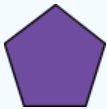6 sides

Hexagon8 sides

OctagonDefinition: Regular Polygon

A shape whose sides have the same length and whose angles have the same measure.

Definition: Irregular Polygon

A shape whose sides differ in length or have angles of different measure.

## Polygon Definitions

Definition: Kite

A quadrilateral with two consecutive sides having equal lengths and the other two sides also have equal lengths.

Definition: Trapezoid

A quadrilateral with at least one pair of opposite sides parallel.

Definition: Isosceles Trapezoid

A trapezoid with both angles next to one of the parallel sides having the same size.

Definition: Parallelogram

A trapezoid with pairs of opposite sides parallel.

Definition: Rectangle

A parallelogram with a right angle.

Definition: Rhombus

A quadrilateral with all sides being the same.

Definition: Square

A rectangle that has four equal sides.

Table 6.2.2: Triangles

Name

Definition

Triangle

SIDES

Equilateral

All three sides are equalIsosceles

Only two sides are equal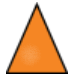Scalene

All three sides are different in lengthANGLES

Acute

Each angle is less than 900Right

One angle is 900Obtuse

One angle is more than 900## Partner Activity 1

Draw the following triangles

1. Isosceles right triangle
2. Scalene obtuse triangle
3. Equilateral right triangle

## Partner Activity 2

1. Is a rectangle a square? Is a square a rectangle?
2. Multiple Choice: Which one is NOT a name for the figure below?
1. Polygon
3. Parallelogram
4. Trapezoid
1. What is the difference between a regular and irregular polygon?

• Angles in a triangle add up to 1800
• An angle forming a straight line is also 1800
• Any quadrilateral (4-sided figure) is 3600
• Angles which round a point add up to 3600
• The two base angles of an isosceles triangle are equal

Why does a triaangle add up to $$180^{\circ }$$

A full circle is $$360^{\circ}$$. Half of a circle, called a semicircle, would then be $$180^{\circ }$$. The diameter (a line which passes through the center of the circle) of the semicircle is then also $$180^{\circ }$$. Therefore, all straight lines are $$180^{\circ }$$. See the figure below. Knowing that all straight lines are $$180^{\circ }$$, we look at the figure below of the line and triangle.

Since a line is $$180^{\circ }$$, we know that angles $$A_1$$, B, and $$C_1$$ must add up to $$180^{\circ }$$. A theorem (proven statement) in Geometry states that alternate (opposite sides) interior angles are congruent (equal). Angles $$A_1$$ and $$A_2$$ are alternate interior, cut by the transversal (line) connecting angle $$A_2$$ to the straight line. Angles $$C_1$$ and $$C_2$$ follow a similar approach.
Since the measures of angles $$A_{1}=A_{2}$$, $$C_{1}=C_{2}$$, and $$A_{1}+B+C_{1}=180$$, then by substitution, $$A_{2}+B+C_{2}=180$$. Therefore, triangle $$A_{2} B C_{2}$$ adds up to $$180^{\circ }$$.

## Partner Activity 3

The sum of the interior angles of any polygon is represented by: $$180(n-2)$$.

1. Find the sum of the interior angles of a triangle, using the formula.
2. Find the sum of the interior angles of a pentagon, using the formula.
3. Find the sum of the interior angles of a 15-sided polygon, using the formula.
4. What is the sum of the EXTERIOR angles of a pentagon?

## Complementary and Supplementary Angles

Definition: Complementary Angles

Complementary angles are any two angles with a sum of 900. See angles C and D below.

Definition: Supplementary Angles

Supplementary angles are any two angles with a sum of 1800. See angles A and B below.

## Partner Activity 4

1. You have two supplementary angles. One angle is 300. What is the measure of the other angle?
2. One angle is complementary to another angle. The first one is 490. What is the measure of the second angle?

## Practice Problems

(Problems 1 – 4) Find the measure of angle b.

1.2.3.4.(Problems 5 – 6) Find the measure of each angle indicated.

1.2.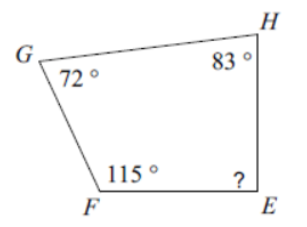(Problems 7 – 10) Classify each angle as acute, obtuse, right or straight.

1. $$121^{\circ}$$

2. $$180^{\circ}$$

3.4.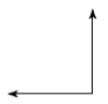(Problems 11 – 12) Classify each triangle by its angles.

1.2.(Problems 13 – 14) Classify each triangle by its angles and sides.

1.2.(Problems 15 – 16) Sketch an example of the type of triangle described.

1. Acute Isosceles

2. Right Obtuse

(Problems 17 – 18) Write the name of each polygon.

1.2.(Problems 19 – 22) Find the interior angle sum for each polygon. Round your answer to the nearest tenth, if necessary.

1.2.3.4.(Problems 23 – 26) State if the polygon is regular or irregular.

1.2.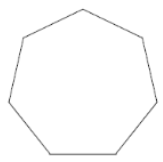3.4.This page titled 7.6: Polygons is shared under a not declared license and was authored, remixed, and/or curated by Amy Lagusker.